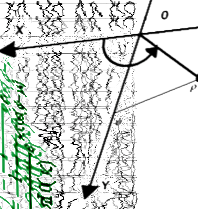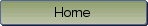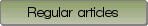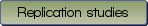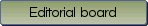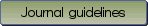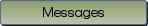Testing the number of required dimensions in exploratory factor analysis

Bibliographic information: BibTEX format RIS format XML format APA style
Cited references information: BibTEX format APA style

Achim, André
64-74
Keywords: Exploratory Factor Analysis , Monte Carlo Comparisons , Number of Factors
(no sample data)   (Appendix)

While maximum likelihood exploratory factor analysis (EFA) provides a statistical test that $k$ dimensions are sufficient to account for the observed correlations among a set of variables, determining the required number of factors in least-squares based EFA has essentially relied on heuristic procedures. Two methods, Revised Parallel Analysis (R-PA) and Comparison Data (CD), were recently proposed that generate surrogate data based on an increasing number of principal axis factors in order to compare their sequence of eigenvalues with that from the data. The latter should be unremarkable among the former if enough dimensions are included. While CD looks for a balance between efficiency and parsimony, R-PA strictly test that $k$ dimensions are sufficient by ranking the next eigenvalue, i.e. at rank $k+1$, of the actual data among those from the surrogate data. Importing two features of CD into R-PA defines four variants that are here collectively termed Next Eigenvalue Sufficiency Tests (NESTs). Simulations implementing 144 sets of parameters, including correlated factors and presence of a doublet factor, show that all four NESTs largely outperform CD, the standard Parallel Analysis, the Mean Average Partial method and even the maximum likelihood approach, in identifying the correct number of common factors. The recommended, most successful NEST variant is also the only one that never overestimates the correct number of dimensions beyond its nominal $\alpha$ level. This variant is made available as R and MATLAB code as well as a complement incorporated in a Microsoft Excel file.

Be informed of the upcoming issues with RSS feed:RSS